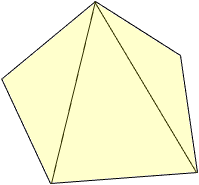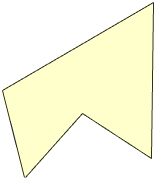Quandaries and Queries Name: ashish Who is asking: Other Level: Middle Question: What is the sum of all the measures of the angles of a Pentagon Hi Ashish, Suppose that the pentagon is concave. Choose one of the vertices and draw the diagonals from the two opposite vertices to subdivide the pentagon into three triangles.The sum of the interior angles of the pentagon is the sum of the interior angles of the three triangles. The sum of the interior angles of a triangle is 180o so the sum of the interior angles of the pentagon is 3 times 180o which is 540o. What if the pentagon is not convex?Penny Go to Math Central